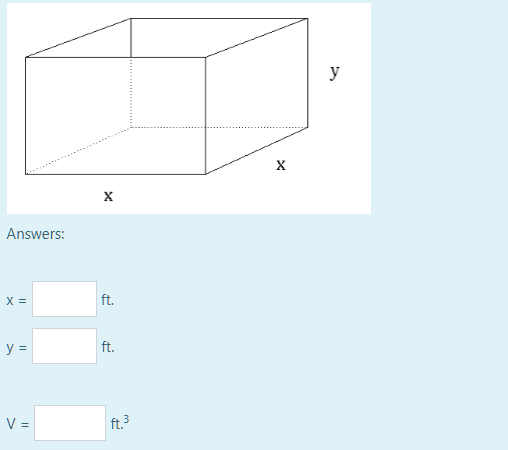x y and z

 Your name to display (optional): Email me at this address if my answer is selected or commented on: Privacy: Your email address will only be used for sending these notifications. Anti-spam verification: To avoid this verification in future, please log in or register.

The area of the 4 sides totals 4xy and the area of the base is x² so the total surface area is 4xy+x²=48, therefore y=(48-x²)/4x. The volume V=x²y=x²(48-x²)/4x=x(48-x²)/4 or 12x-x³/4. dV/dx=12-3x²/4=0 when V is max or min.

So 3x²/4=12, x²=16, x=4ft (reject x=-4). Therefore y=(48-16)/16=2ft. Volume is 32 cu ft.

by Top Rated User (1.0m points)